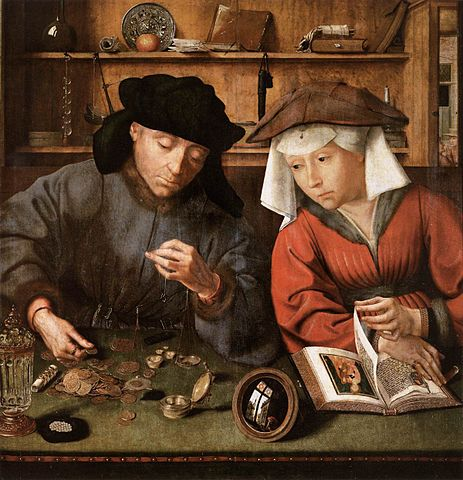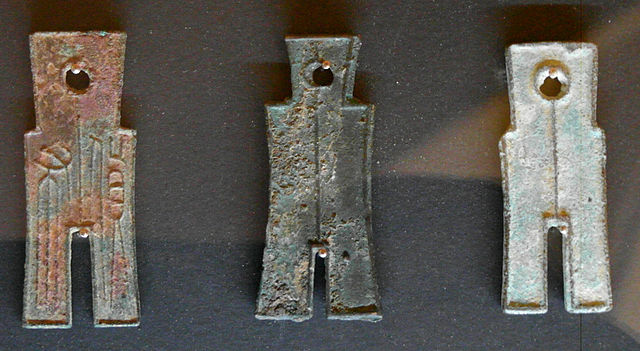I think most people make change for amounts of money using a greedy algorithm: repeatedly start with an empty pile of money, and repeatedly add the largest-valued coin or bill that keeps the value of the pile at or under the desired total, until the total is reached. This can use more coins than necessary, though, for exotic coinage systems, or even some less-exotic ones like US coins without the nickels.There’s a simple test for whether the greedy algorithm is always optimal for a system of coins. It’s called the one-point test, and was first published by Magazine, Nemhauser, and Trotter in 1975. It actually tests a stronger property, whether every prefix of the sequence is optimal for the greedy algorithm. To perform this test, look at each consecutive pair of coin values $$x$$ and $$y$$. Round $$y$$ up to a multiple $$kx$$ of $$x$$. Then we could make change for $$kx$$ non-greedily, using $$k$$ copies of the $$x$$ coin. Does the greedy algorithm do at least as well, using $$y$$ and some smaller coins? If not, then we have found an example where greedy is non-optimal. But if a coin system passes this test for all of its coins, then the whole sequence and all of its prefixes are greedy-optimal.

This immediately shows that any sequence of coin values where each is an integer multiple of the next is greedy-optimal, because the greedy algorithm will always represent each tested value $$kx$$ with the single coin $$y$$. And you can check that it’s true for US money as well (with the nickel, and with or without the \$2 bill). But the no-nickel sequence $$1,10,25$$ already fails the test, because when $$x=25$$ and $$y=10$$, $$ky=30$$ is not represented optimally by the greedy algorithm.Han Dynasty spade coins were not very round

The one-point test also shows that some systems of money that do not involve round multiples are still greedy-optimal. For instance, in the Fibonacci Republic they use coins of $$1, 2, 3, 5, 8, 13, 21, 34,$$ and $$55$$ cents, a Fibonacci dollar coin of $$89$$ cents, and bills for the larger denominations of $$144$$ cents etc. Each of these numbers is at most double the next, and we can represent each doubled Fibonacci number $$ky=2F_i$$ in the one-point test greedily using the identity $$2F_i=F_{i+1}+F_{i-2}.$$ The nearby country of Mersenneland instead uses the numbers $$M_i=2^i-1=1,3,7,15,31,\dots$$ for their money. Each is more than double but at most triple the next, and we can represent each number $$ky=3M_i$$ in the one-point test greedily using the identity $$3M_i=M_{i+1}+2M_{i-1}$$.

Regardless of whether a coinage system is greedy-optimal or not, one can use another simple algorithm to find the amounts of money that would cause the greedy algorithm the most trouble. Suppose that the sequence of coins is $$c_i$$, and we want to calculate the smallest amount of money $$r_i,$$ that causes the greedy algorithm to use $$i$$ coins. Then $$r_{i+1}=r_i+c_j,$$ where $$c_j$$ is the smallest coin value such that $$r_i+c_j\lt c_{j+1}.$$ That is, we look for the first gap larger than $$r_i$$ in the sequence of coin values, and we add $$r_i$$ to the smaller of the two coins forming this gap.

In Factoria, the coin values are $$1,2,6,24,$$ and $$120$$ cents (the Factorial dollar), and they have bills for $$6, 42, 336\dots$$ dollars. This is a greedy-optimal coin system, because each coin or bill is an integer multiple of its predecessor. Plugging in the factorial numbers to the formula above, the numbers that are hardest to express as sums of factorials (whether greedily or in any other way) are

$$1, 3, 5, 11, 17, 23, 47, 71, 95, 119, 239, 359, \dots$$ (OEIS A200748).

In the breakaway republic of South Factoria, they’ve long been familiar with how hard it is to make change using factorials. So, wanting a currency that makes what was once difficult more easy, they use the numbers in A200748 as the values of their own money. This choice also has the advantage that it’s difficult to exchange South Factorial money for Factorial money. But is this a greedy-optimal coin system?

To see this, we need a clearer idea of how the numbers in A200748 are constructed. Each one is a factorial plus its predecessor. A straightforward induction using the formulas for $$c_i$$ and $$r_i$$ shows that, for each integer $$i$$, there are exactly $$i$$ differences equal to $$i!$$, running from $$i!-1$$ to $$(i+1)!-1$$. With this pattern in hand, we can show that sequence A200748 again passes the one-point test. When the sequence jumps from $$i!-1$$ to $$2i!-1$$, the one-point test runs the greedy algorithm on $$3(i!-1)=(2i!-1)+(i!-1)+1$$, which it passes. And for any other jump in the sequence, from $$xi!-1$$ to $$(x+1)i!-1$$ for some integer $$x\gt 1,$$ the one-point test runs the greedy algorithm on $$2(xi!-1)=((x+1)i!-1)+((x-1)i!-1),$$ again passing. So South Factorial money is indeed greedy-optimal.

Which numbers are hard to change in South Factoria? As before we can construct the sequence recursively, by adding the starting points of big gaps to previous values in the sequence. This gives us the numbers

$$1, 2, 7, 30, 149, 868, 5907, 46226, 409105,\dots$$ (OEIS A136574).

This construction also gives us a new insight into number sequence A136574, which was originally defined in a more complicated way as the row sums of a triangle of numbers involving factorials. Remember that that each term in this sequence is the previous term plus the smallest coin that comes before a big gap. And in the hard-to-change-factorially numbers A200748, the smallest coin that comes before a big gap is always one less than a factorial. So this gives us the nice recurrence $$a(i)=a(i-1)+i!-1$$ for the values of A136574.

So far, the systems of coins we’ve been considering are all pretty sparse. A dense system of coins (one with many coin values) will have a sparse system of hard-to-represent values, and vice versa. In the limit, for a system of coins with bounded gap size, the sequence of hard-to-represent values will be finite, and conversely for a system of finitely many coins the hard-to-represent values will be eventually periodic. So, to conclude with just a couple more examples, of greater density: if the coin values are all the square numbers (not greedy-optimal: $$12 = 3\times 4 = 9 + 1 + 1 + 1$$ fails the one-point test) then the hard-to-represent-greedily values are

$$1, 2, 3, 7, 23, 167, 7223, 13053767,\dots$$ (OEIS A006892).

For instance, even though every number can be represented as a sum of four squares, the greedy algorithm uses five for $$23 = 16 + 4 + 1 + 1 + 1$$. And similarly, for prime-number coinage (plus a one-cent coin, again not greed-optimal), the hard-to-represent values are the Pillai sequence

$$1, 4, 27, 1354, 401429925999155061,\dots$$ (OEIS A066352).

To find the next term in the sequence, we need to find the first prime gap bigger than 401429925999155061. As the OEIS entry states, reaching a gap this big is likely to require hundreds of millions of digits. So in practice, all reasonable amounts of change require only four coins or bills in the prime number coinage system, even when we make change greedily. Goldbach’s conjecture suggests, though, that a more clever change-making strategy would need only three coins for every value. So greed is not always good.(G+)